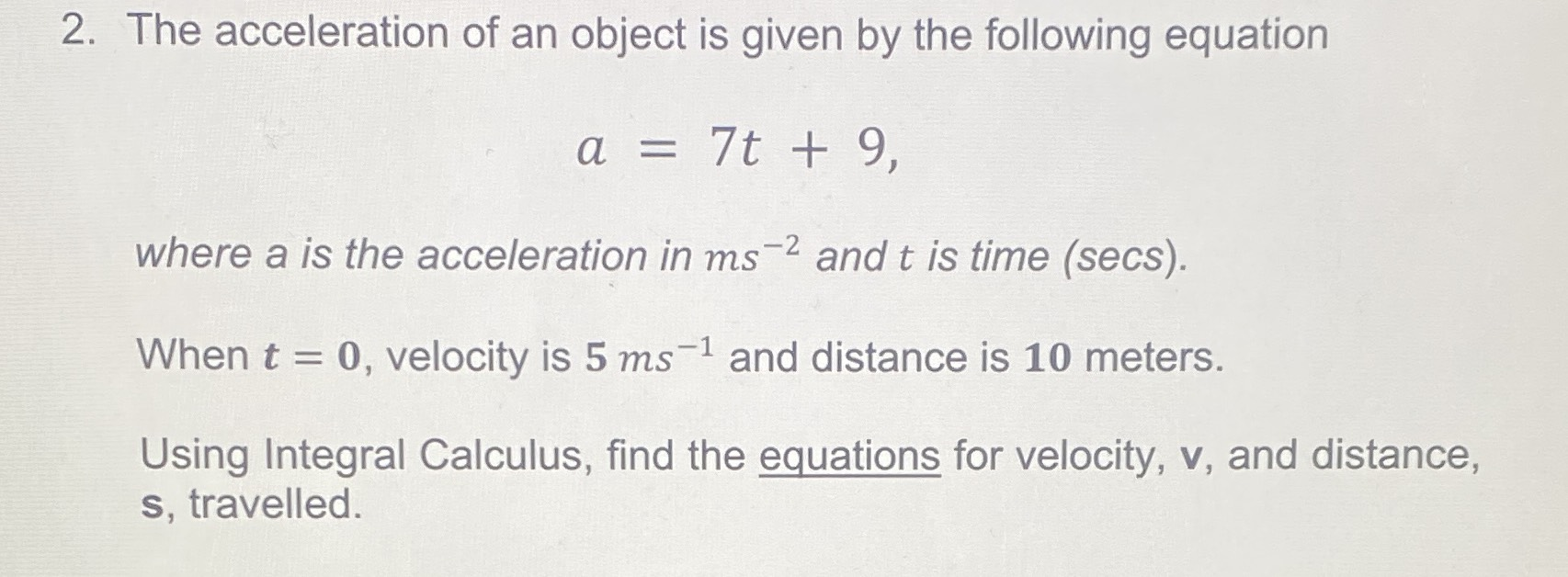### ¿Todavía tienes preguntas de matemáticas?

Pregunte a nuestros tutores expertos
Algebra
Pregunta

2. The acceleration of an object is given by the following equation

$$a = 7 t + 9$$ ,$$\frac{dv}{dt} = 7t+ 9 , v = \frac{7t^2}{2} + 9t+ 5$$ , $$\frac{ds}{dt}= \frac{7t^2}{2}+ 9t+ 5 ,s = \frac{7t^3}{6} + \frac{9t^2}{2} + 5t+ 10$$